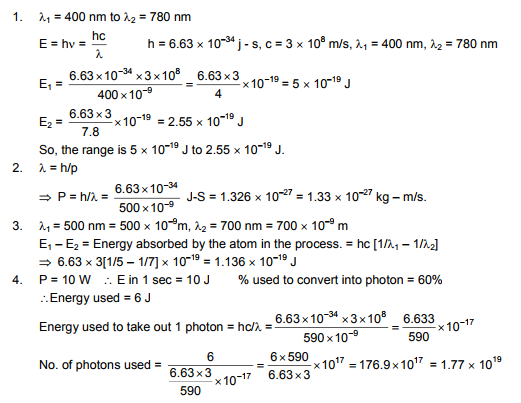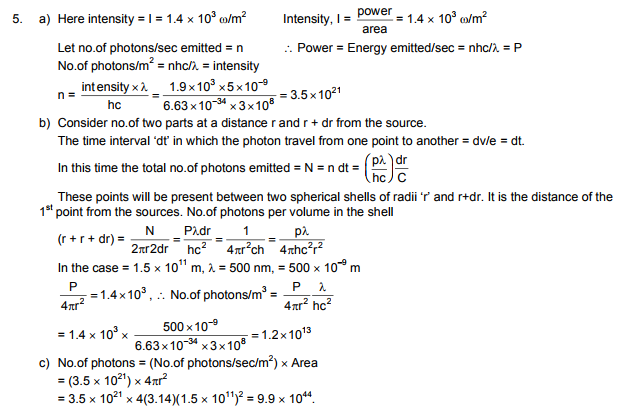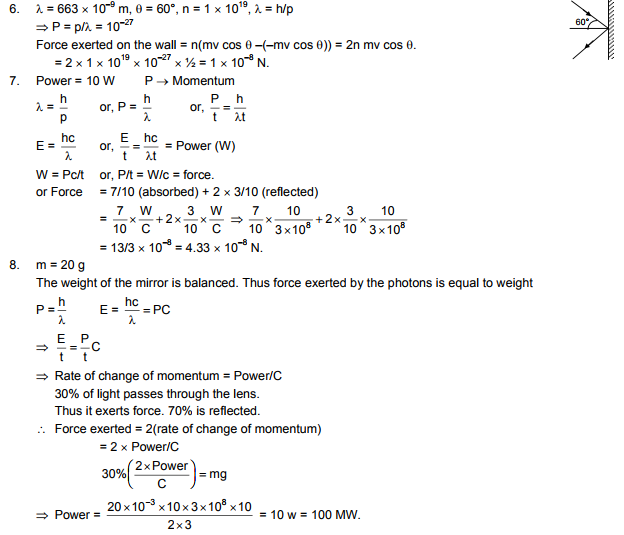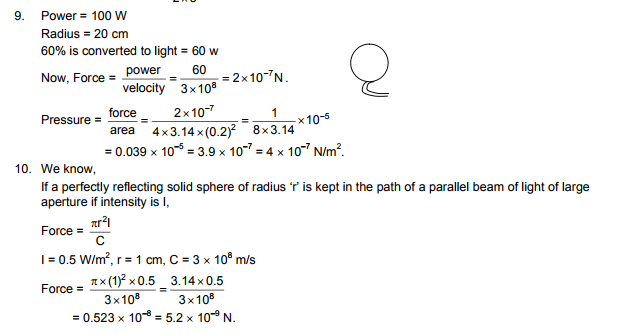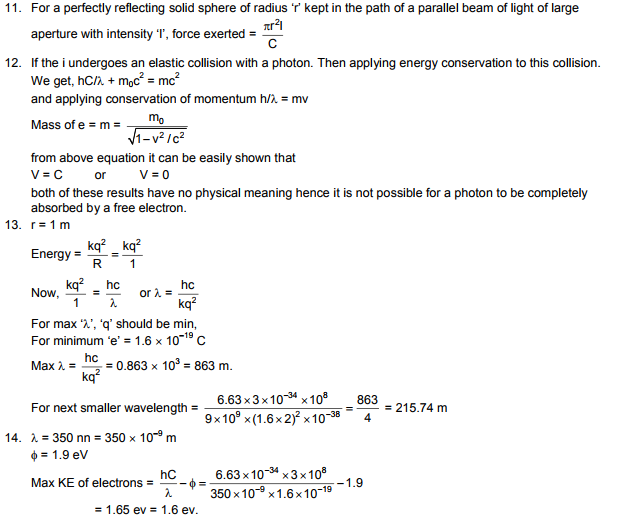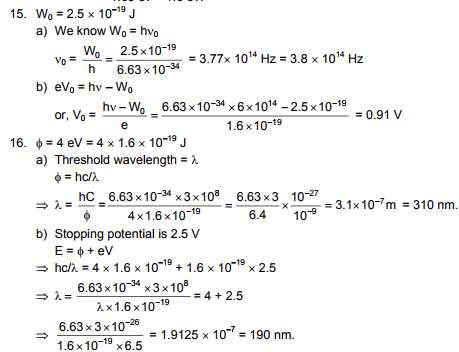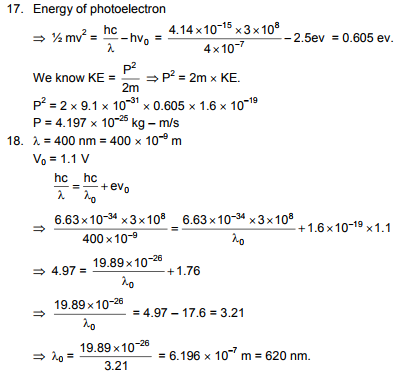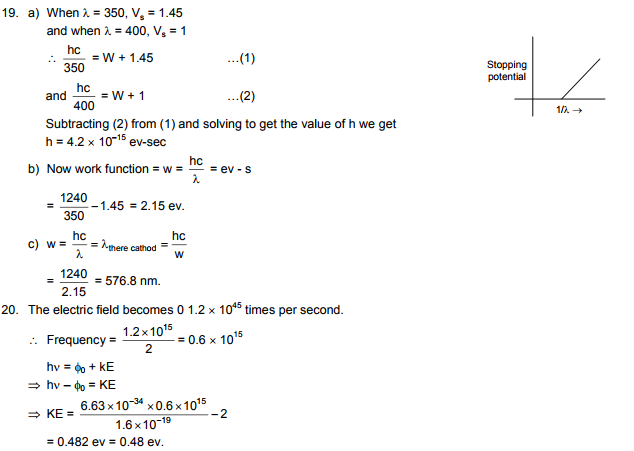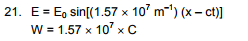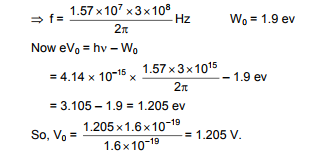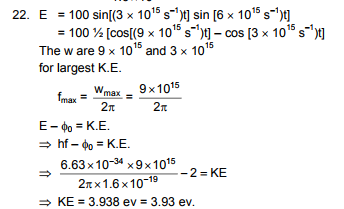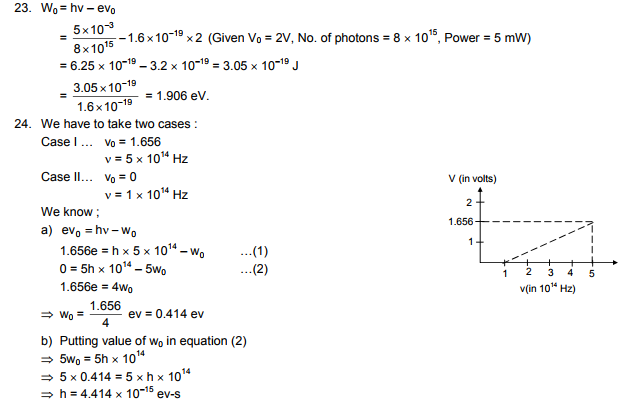# HC Verma Solutions Vol 2 Chapter 20 Photoelectric Effect

HC Verma Solutions Vol 2 Chapter 20 Photoelectric Effect is a premier study tool for students who are looking to develop a fresh approach in solving questions given in this chapter. The solutions cover all the exercises and topics such as energy absorbed by a particle when the wavelength is given, etc.  There are problems such as:

• Finding the energy to take out one photon along with the intensity and also have problems related to counting the number of photons.
• Questions related to momentum, force, pressure, and problems in which you have to find the rate of change of momentum.
• We will be solving questions based on the law of conservation of energy in which you will be provided with kinetic energy, threshold wavelength, stopping potential, etc.
• We will be seeing questions on finding the work function when frequency and the electric field are given.

These questions which are dealt comprehensively in the HC Verma solutions will help students understand this chapter in a better way.

## Key Concepts Covered In Photoelectric Effect Chapter

Some of the other key topics discussed in this unit include;

• Photon Theory of Light
• Photoelectric Effect
• Matter Waves

## Important Questions In Chapters 20

1. Is it possible to deflect a photon using an electric field or a magnetic field?

2. One of the properties of an electron is that it has a wavelength. Does it also have a colour? Elaborate.

3. What will happen to the stopping potential if the frequency of light in a photoelectric experiment is doubled?

(a) become more than double (b) doubled (c) become less than double (d) halved

4. State the condition where the equation E = pc becomes valid.

(a) for both an electron and a photon (b) for an electron (c) for a photon (d) neither for an electron or photon

5. Why does the photoelectric effect support the quantum nature of light?

(a) Because there is a minimum frequency below which no photoelectrons are emitted.
(b) the maximum kinetic energy of photoelectrons depends only on the frequency of light and not on its intensity.
(c) the photoelectrons leave the surface immediately even when the metal surface is faintly illuminated.
(d) The electric charge of the photoelectrons is quantized.

## HC Verma Solutions Vol 2 Photoelectric Effect Chapter 20